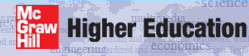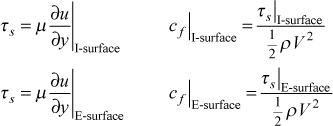# Institut für Thermodynamik der Luft- und Raumfahrt - Universität StuttgartMechanical Engineering - The University of Texas at Austin# friction

Friction Coefficient - Newton's law of viscosity is used to formulate the surface shear stress for each surface that is a no-slip surface, and the friction coefficient is the classical Fanning friction coefficient.For the wall shear stress the dynamic viscosity is evaluated at the surface temperature and for the friction coefficient the fluid density is evaluated at the mean temperature.

Note:The classical Moody friction factor for a pipe is f = 4 cf  (which leads to the familiar f=64/Re result for laminar flow in a smooth pipe, whereas cf =16/Re). The friction factor is typically used in engineering pressure drop calculations. For more information on the differences, see Chapter 7 of CHMT.

website updated Sept 2010   © 1996-2010 Michael E. Crawford - all rights reserved - website validated for CSS 2.1 and XHTML 1.0 strict at www.w3.org